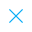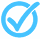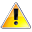Call Support +91-85588-96644

Keep me logged in
You can't leave Captcha Code empty
By submitting this form, you agree to the Terms & Privacy Policy.
ORTests given# Linear Equations in two variables Test - 2 By TCY All tests

Questions

Time

Highest
score

Level

## ENGLISH

Language

Topics Covered:Linear Equations and Simultaneous EquationsLinear Equations in Two VariablesMathematicsPair of Linear Equations in Two VariablesSolution of Problems by EliminationSolving by EliminationAlgebraic SolutionsLinear Equation in 2 VariablesLinear Equations in 2 Variables